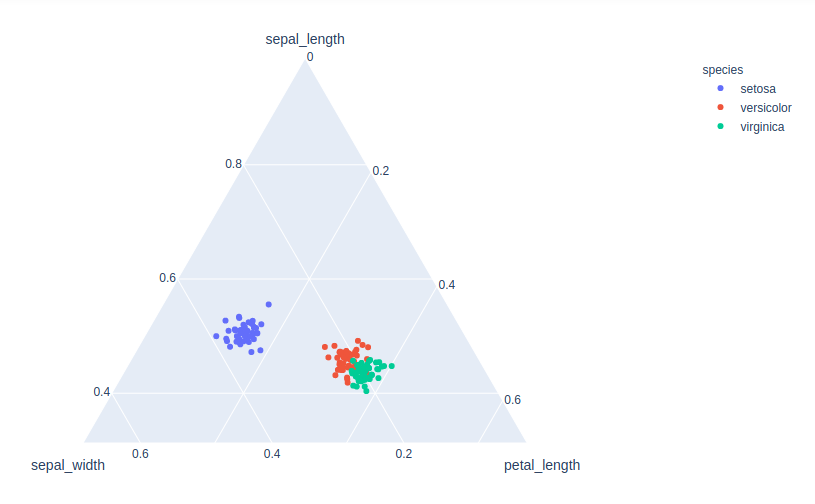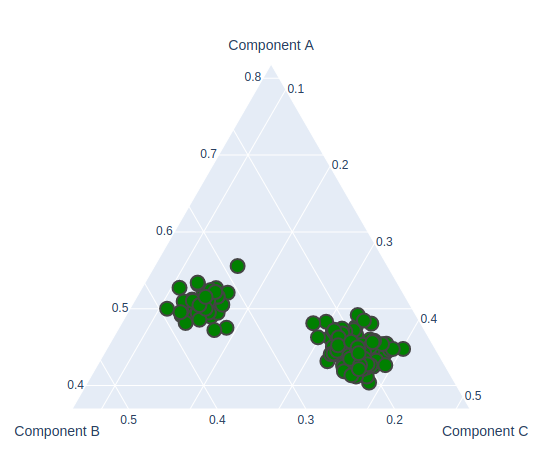Open in App
Not now

# Ternary Plots in Plotly

• Last Updated : 01 Oct, 2020

A Plotly is a Python library that is used to design graphs, especially interactive graphs. It can plot various graphs and charts like histogram, barplot, boxplot, spreadplot, and many more. It is mainly used in data analysis as well as financial analysis. plotly is an interactive visualization library.

## Ternary Plots in Plotly

A ternary plot is also known as a ternary graph, triangle plot, simplex plot which is a barycentric plot on three variables which sum to a constant. It graphically depicts the ratios of the three variables as positions in an equilateral triangle. It can be created using two-class i.e. express class and graph_objects class.

### Using express class

In plotly, express is an easy-to-use,high-level interface that helps to operates a variety of types of data and produce simple style figures. It provides scatter_ternary() method to create ternary plots.

Syntax: scatter_ternary( a=None, b=None, c=None, color=None,labels={},width=None, height=None)

Parameters:

a: Values from this column or array_like are used to position marks along the a axis in ternary coordinates.

b: Values from this column or array_like are used to position marks along the b axis in ternary coordinates.

c: Values from this column or array_like are used to position marks along the c axis in ternary coordinates.

color:  Either a name of a column in data_frame, or a pandas Series or array_like object. Values from this column or array_like are used to assign color to marks.

width: The figure width in pixels.

height: The figure height in pixels.

Example:

## Python3

 `import` `plotly.express as px`` ` ` ` `df ``=` `px.data.iris()``fig ``=` `px.scatter_ternary(df, a``=``"sepal_length"``,``                         ``b``=``"sepal_width"``, c``=``"petal_length"``,``                         ``color``=``"species"``, size_max``=``20``)``fig.show()`

Output:### Using Graph_Objects class

Let’s see one example of a ternary scatter plot with plotly graph objects to make it clear. It can be created using the Scatterternary() method.

Example:

## Python3

 `import` `plotly.express as px``import` `plotly.graph_objects as go`` ` ` ` `df ``=` `px.data.iris()``fig ``=` `go.Figure(go.Scatterternary({``    ``'mode'``: ``'markers'``,``    ``'a'``: df[``'sepal_length'``],``    ``'b'``: df[``'sepal_width'``],``    ``'c'``: df[``'petal_length'``],``    ``'marker'``: {``        ``'color'``: ``'green'``,``        ``'size'``: ``14``,``        ``'line'``: {``'width'``: ``2``}``    ``}``}))`` ` `fig.show()`

Output:My Personal Notes arrow_drop_up